### Home > A2C > Chapter 6 > Lesson 6.2.3 > Problem6-89

6-89.
1. Graph the two functions below on the same set of axes. Homework Help ✎ y = 3(2x)
y = 3(2x) + 10

1. How do the two graphs compare?

2. Suppose the first equation is y = kmx and the graph is shifted up b units. What is the new equation?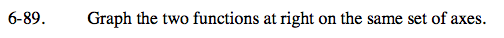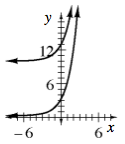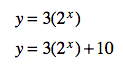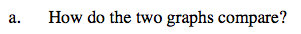Look at their y-intercepts.

The second graph is the first, but shifted 10 units upward.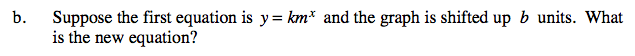See part (a).